# 特征工程学习，19项实践Tips！代码已开源！

GitHub上有一个专门针对特征工程技巧的“锦囊”，叫做《Tips-of-Feature-engineering》，作者把网路上、书本上的一些特征工程的项目，抽取其中的挖掘技巧，并把这些小技巧打包成一个又一个的小锦囊，供大家去检索并且学习，还蛮不错的！

https://github.com/Pysamlam/Tips-of-Feature-engineering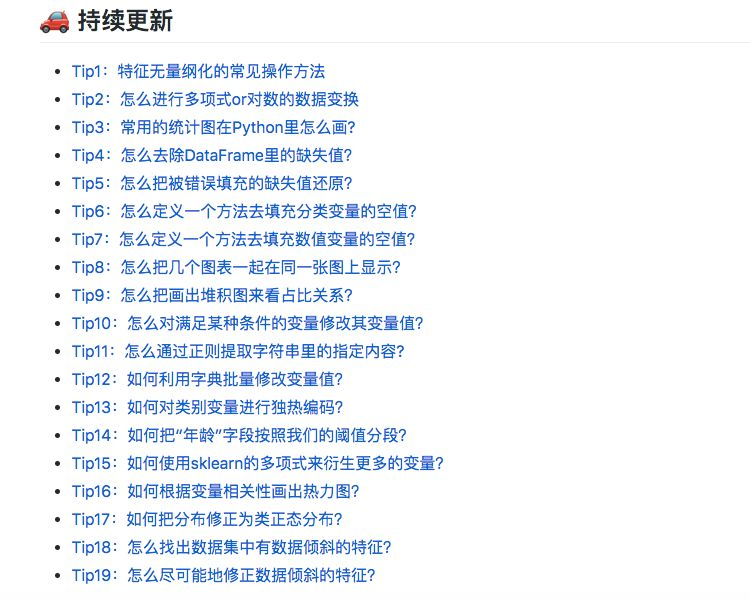### Tip8：怎么把几个图表一起在同一张图上显示？

#### 关键代码

import matplotlib.pyplot as plt

# 设置figure_size尺寸
plt.rcParams['figure.figsize'] = (8.0, 6.0)

fig = plt.figure()

# 设定图表颜色
fig.set(alpha=0.2)

# 第一张小图
plt.subplot2grid((2,3),(0,0))
data_train['Survived'].value_counts().plot(kind='bar')
plt.ylabel(u"人数")
plt.title(u"船员获救情况 (1为获救)")

# 第二张小图
plt.subplot2grid((2,3),(0,1))
data_train['Pclass'].value_counts().plot(kind="bar")
plt.ylabel(u"人数")
plt.title(u"乘客等级分布")

# 第三张小图
plt.subplot2grid((2,3),(0,2))
plt.scatter(data_train['Survived'], data_train['Age'])
plt.ylabel(u"年龄")
plt.grid(b=True, which='major', axis='y')
plt.title(u"按年龄看获救分布 (1为获救)")

# 第四张小图，分布图
plt.subplot2grid((2,3),(1,0), colspan=2)
data_train.Age[data_train.Pclass == 1].plot(kind='kde')
data_train.Age[data_train.Pclass == 2].plot(kind='kde')
data_train.Age[data_train.Pclass == 3].plot(kind='kde')
plt.xlabel(u"年龄")
plt.ylabel(u"密度")
plt.title(u"各等级的乘客年龄分布")
plt.legend((u'头等舱', u'2等舱',u'3等舱'),loc='best')

# 第五张小图
plt.subplot2grid((2,3),(1,2))
data_train.Embarked.value_counts().plot(kind='bar')
plt.title(u"各登船口岸上船人数")
plt.ylabel(u"人数")
plt.show()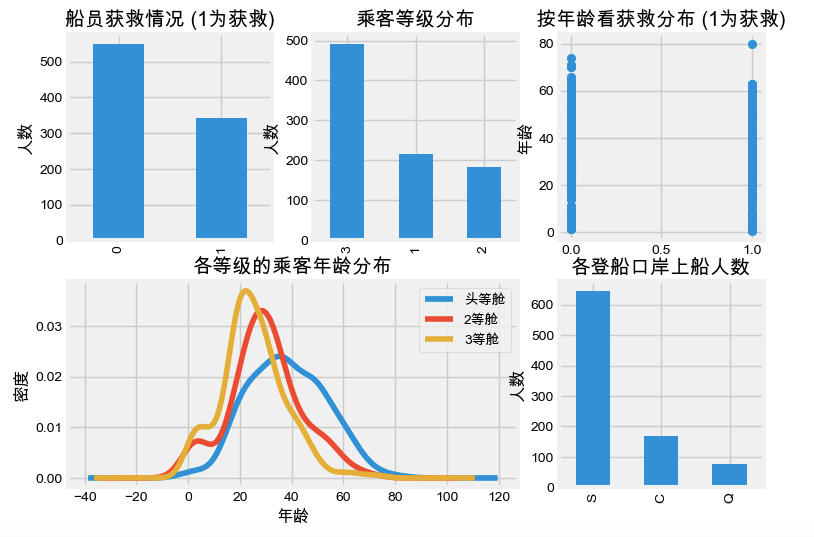### Tip15：如何使用sklearn的多项式来衍生更多的变量？

#### 关键代码

# 扩展数值特征
from sklearn.preprocessing import PolynomialFeatures

x = df[['x','y','z']]
y = df['activity']

poly = PolynomialFeatures(degree=2, include_bias=False, interaction_only=False)

x_poly = poly.fit_transform(x)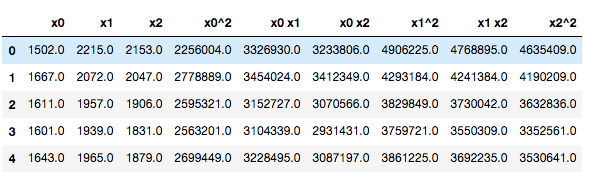### Tip17：如何把分布修正为类正态分布？import pandas as pd
import numpy as np
# Plots
import seaborn as sns
import matplotlib.pyplot as plt

# 读取数据集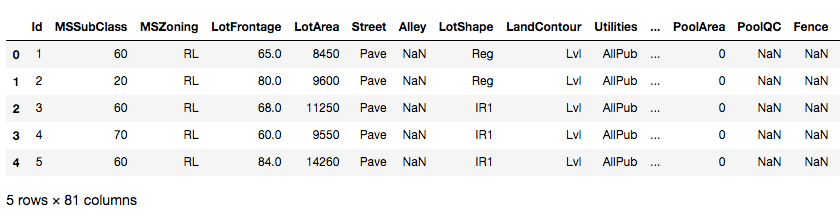sns.set_style("white")
sns.set_color_codes(palette='deep')
f, ax = plt.subplots(figsize=(8, 7))
#Check the new distribution
sns.distplot(train['SalePrice'], color="b");
ax.xaxis.grid(False)
ax.set(ylabel="Frequency")
ax.set(xlabel="SalePrice")
ax.set(title="SalePrice distribution")
sns.despine(trim=True, left=True)
plt.show()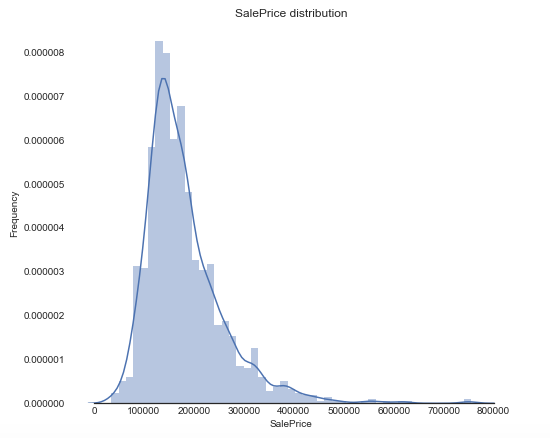# log(1+x) 转换
train["SalePrice_log"] = np.log1p(train["SalePrice"])

sns.set_style("white")
sns.set_color_codes(palette='deep')
f, ax = plt.subplots(figsize=(8, 7))

sns.distplot(train['SalePrice_log'] , fit=norm, color="b");

# 得到正态分布的参数
(mu, sigma) = norm.fit(train['SalePrice_log'])

plt.legend(['Normal dist. ($\mu=$ {:.2f} and $\sigma=$ {:.2f} )'.format(mu, sigma)],
loc='best')
ax.xaxis.grid(False)
ax.set(ylabel="Frequency")
ax.set(xlabel="SalePrice")
ax.set(title="SalePrice distribution")
sns.despine(trim=True, left=True)

plt.show()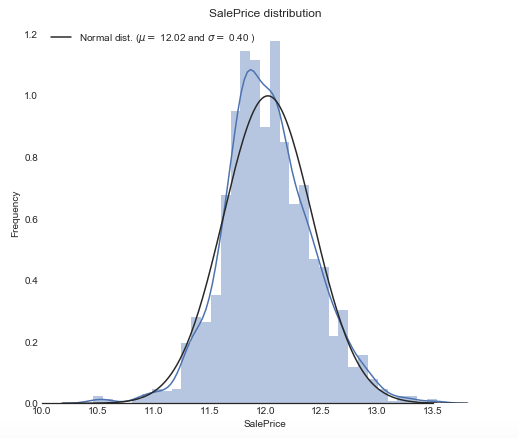https://github.com/Pysamlam/Tips-of-Feature-engineering

AI学习路线和优质资源，在后台回复"AI"获取©️2019 CSDN 皮肤主题: 大白 设计师: CSDN官方博客